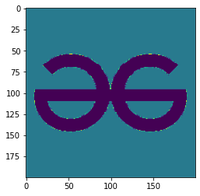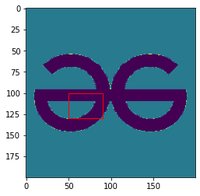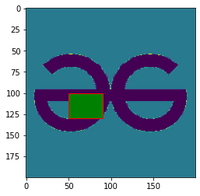Related Articles

# How to Draw Rectangle on Image in Matplotlib?

• Last Updated : 17 Dec, 2020

Prerequisites: Matplotlib

Given an image the task here is to draft a python program using matplotlib to draw a rectangle on it. Matplotlib comes handy with rectangle() function which can be used for our requirement.

Syntax: Rectangle(xy, width, height, angle=0.0, **kwargs)

Parameters:

• xy: Lower left point to start the rectangle plotting
• width : width of the rectangle
• height: Height of the rectangle.
• angle: Angle of rotation of the rectangle.

### Approach

• Import the necessary libraries.
• Insert and display the image.
• Create the figure and axes of the plot
• Add the patch to the Axes
• Display the image

Example 1: Draw a rectangle on an image

## Python3

 `import` `matplotlib.pyplot as plt``import` `matplotlib.patches as patches``from` `PIL ``import` `Image``import` `numpy as np`` ` `x ``=` `np.array(Image.``open``(``'geek.png'``), dtype``=``np.uint8)``plt.imshow(x)`` ` `# Create figure and axes``fig, ax ``=` `plt.subplots(``1``)`` ` `# Display the image``ax.imshow(x)`` ` `# Create a Rectangle patch``rect ``=` `patches.Rectangle((``50``, ``100``), ``40``, ``30``, linewidth``=``1``,``                         ``edgecolor``=``'r'``, facecolor``=``"none"``)`` ` `# Add the patch to the Axes``ax.add_patch(rect)``plt.show()`

Output:original imageimage with rectangle

Example 2: Draw a filled rectangle

## Python3

 `import` `matplotlib.pyplot as plt``import` `matplotlib.patches as patches``from` `PIL ``import` `Image``import` `numpy as np`` ` `x ``=` `np.array(Image.``open``(``'geek.png'``), dtype``=``np.uint8)``plt.imshow(x)`` ` `# Create figure and axes``fig, ax ``=` `plt.subplots(``1``)`` ` `# Display the image``ax.imshow(x)`` ` `# Create a Rectangle patch``rect ``=` `patches.Rectangle((``50``, ``100``), ``40``, ``30``, linewidth``=``1``,``                         ``edgecolor``=``'r'``, facecolor``=``"g"``)`` ` `# Add the patch to the Axes``ax.add_patch(rect)``plt.show()`

Output:original imageimage with rectangle

Attention geek! Strengthen your foundations with the Python Programming Foundation Course and learn the basics.

To begin with, your interview preparations Enhance your Data Structures concepts with the Python DS Course. And to begin with your Machine Learning Journey, join the Machine Learning – Basic Level Course

My Personal Notes arrow_drop_up